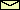# The Horizontally Driven Pendulum

acceleration of gravity = 9.81 m/sec2
Either your Web browser isn't able to run Java applets or you have forgotten to switch on this feature.

For instructions click on here.
For the equation of motion click on here

Suggestions for EXPERIMENTS
1. Choose length = 1 m, damping = 1 sec-1, and amplitude = 0.2 m (this are the default settings when the applet is started). Now, change the frequency of driving very slowly from 0.2 Hz to 0.8 Hz. Observe the change in the amplitude of oscillation and the change in the phase between driving and pendulum oscillations. You can measure it by using the oscilloscope.
 Related topics in the lecture room: Resonance.
2. Choose the parameters as above but now change the frequency between 3 Hz to 4 Hz. Observe a shift of the center of oscillation either to the left or to the right (this is a pitchfork bifurcation). This bifurcation does not occur for the pendulum driven by a periodic force. Why not?
 Related topics in the lecture room: The upside-down pendulum and the harmonic oscillator.
3. Choose length = 1 m, damping = 1 sec-1, amplitude = 0.85 m, and frequency = 1 Hz. You will observe an irregular motion called deterministic chaos with a seemingly random number of left and right turns. On the oscilloscope select the angle as the x-axis and the angular velocity as the y-axis. For the scaling of the axes, choose 180 and 1000, respectively. Now, switch the oscilloscope on and you will be fascinated by the beautyful curves drawn on the screen. Turning the oscilloscope into a Poincaré map by selecting external triggering in order to see the irregularity better.

© 1998 Franz-Josef Elmer,Franz-Josef doht Elmer aht unibas doht ch, last modified Saturday, June 27, 2011.